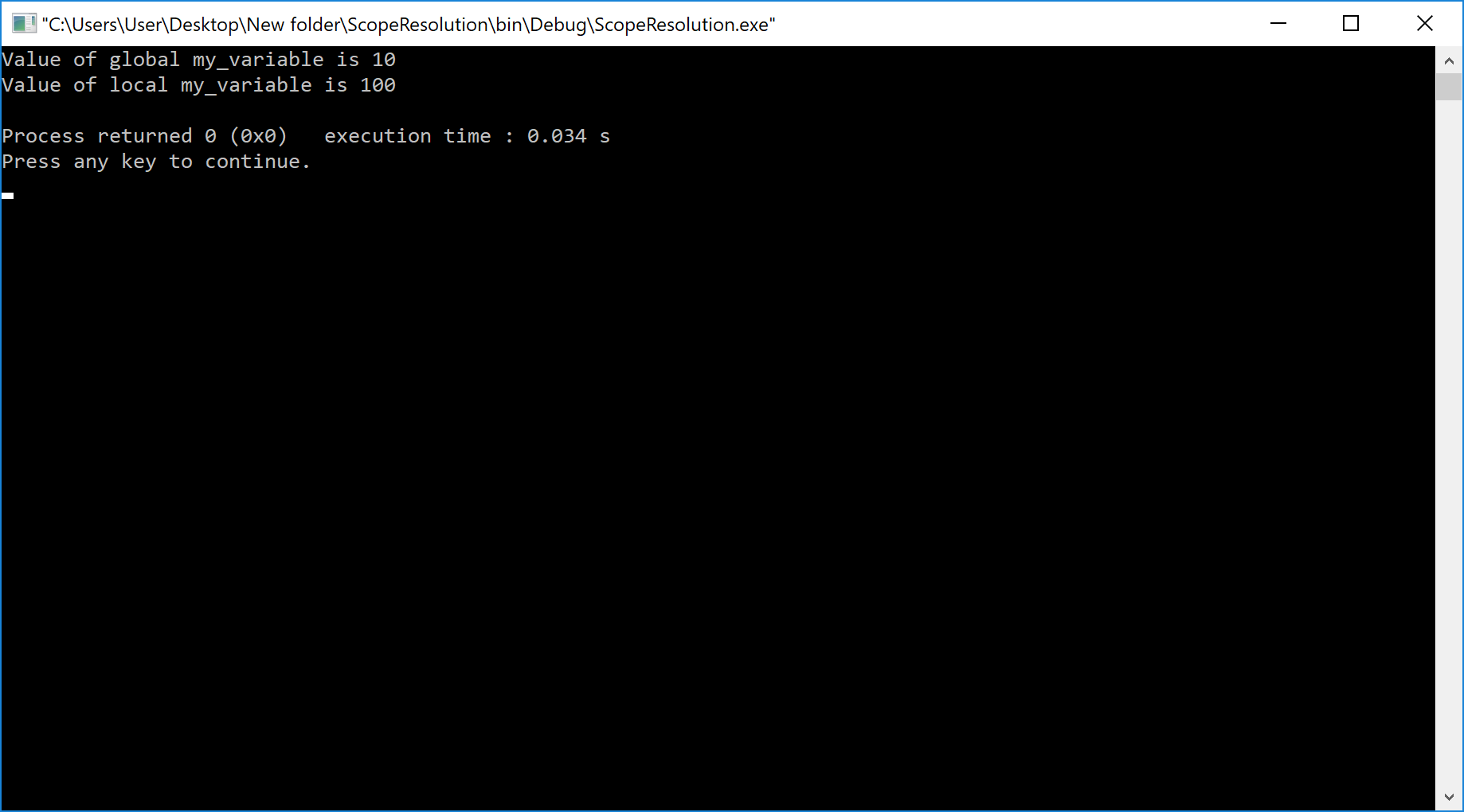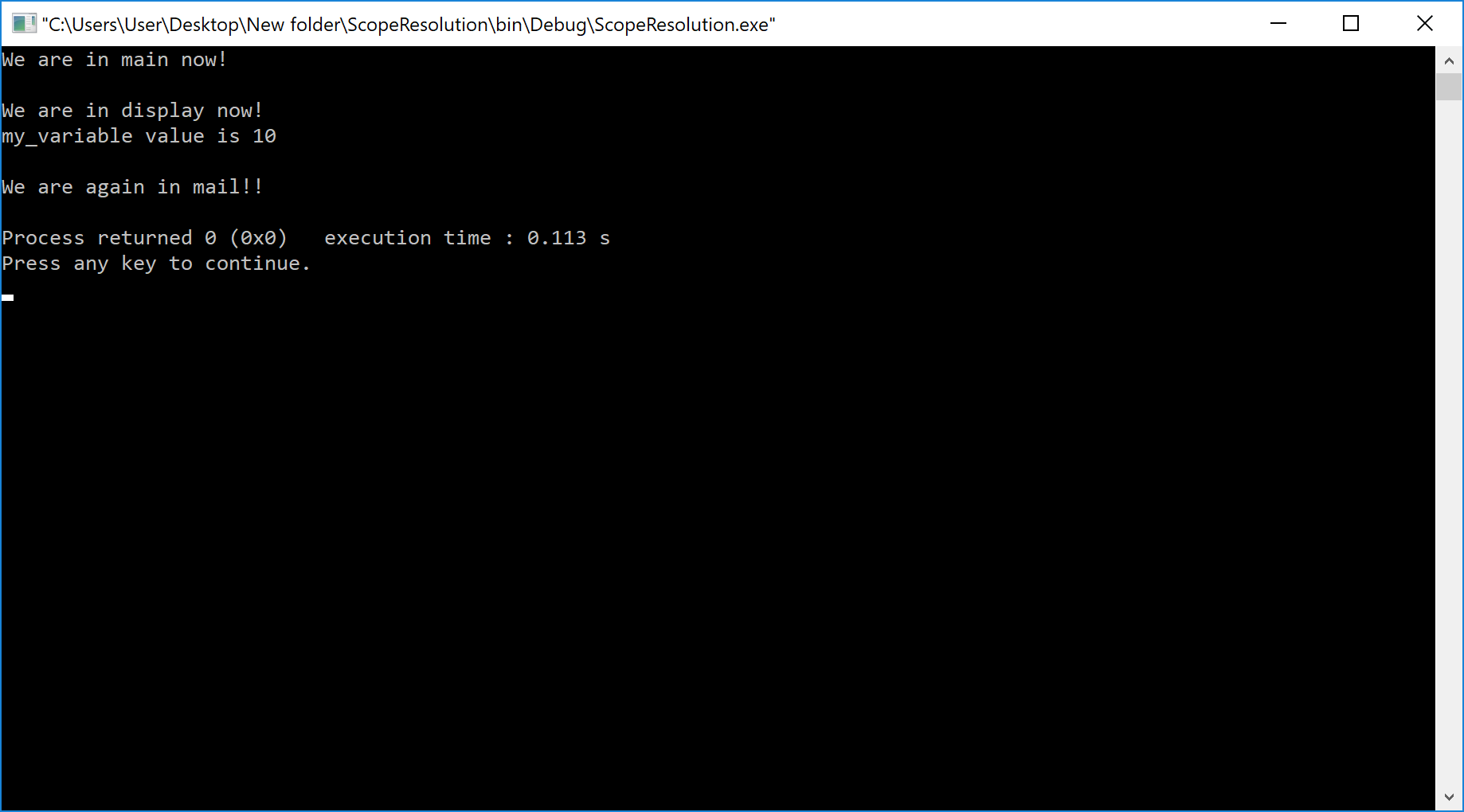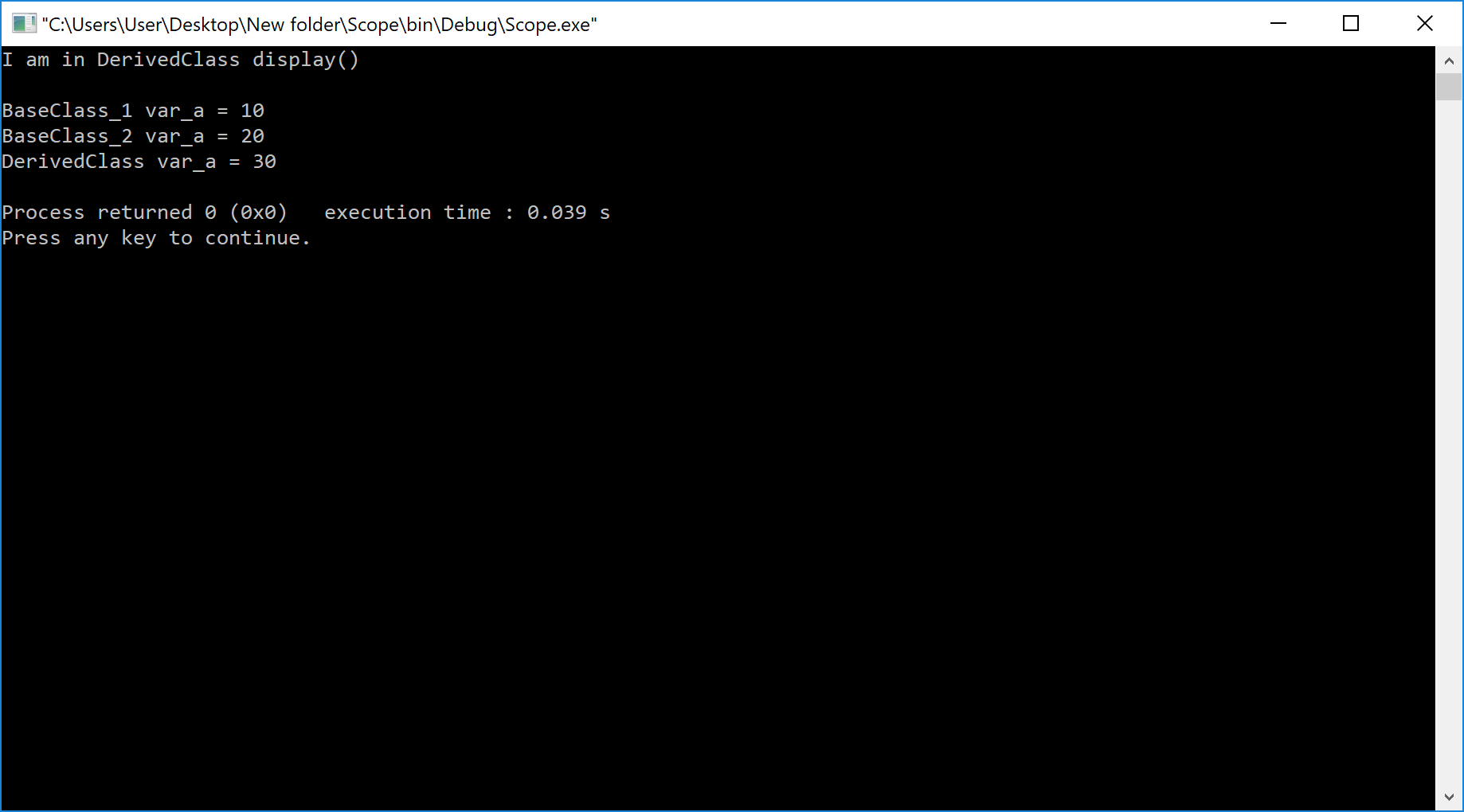The perfect place for easy learning...

×

# Aim

Write a C++ program to use scope resolution operator. Display the various values of the same variables declared at different scope levels.

# Solution

The scope resolution operator is used to access the hidden names which are at different scope levels. In C++, the scope resolution operator has the following uses.

# ⇢ Accessing Global variable

When both global and local variables have the same name then global variable in hidden inside the local scope. Here, we use the scope resolution operator to refer to the global variable.

Example
``````#include <iostream>
using namespace std;

int my_variable = 10;  // Global variable my_variable

int main()
{
int my_variable = 100; // Local variable my_variable

cout << "Value of global my_variable is " << ::my_variable << endl;
cout << "Value of local my_variable is " << my_variable << endl;

return 0;
}
``````

# Result# ⇢ Defining a function out of class

When a class has a function prototype inside but the definition is outside of the class. Here, to define the function outside the class we use the scope resolution operator.

Example
``````#include <iostream>

using namespace std;

class My_Class
{
public:
int my_variable = 10;
void display(); //Prototype of display function
};

void My_Class :: display(){ //Defining function using Scope Resolution Operator
cout << endl << "We are in display now!" << endl;
cout << "my_variable value is " << my_variable << endl << endl;
}

int main(){

My_Class obj;
cout << "We are in main now << endl;
obj.display();
cout << "We are again in mail!!" << endl;

return 0;
}
``````

# Result# ⇢ Accessing static members of a class

The scope resolution operator can be used to access static members of a class when there is a local variable with the same name.

Example
``````#include <iostream>

using namespace std;

class StaticTest
{
static int x;
public:
static int y;

// Local parameter 'x' hides class member
// 'x', but we can access it using ::
void my_function(int x)
{
// We can access class's static variable
// even if there is a local variable
cout << "Value of static x is " << StaticTest::x;

cout << "\nValue of local x is " << x;
}
};

// In C++, static members must be explicitly defined
// like this
int StaticTest::x = 1;
int StaticTest::y = 2;

int main()
{
StaticTest obj;
int x = 3 ;
obj.my_function(x);

cout << "\n\nStaticTest::y = " << StaticTest::y << endl;

return 0;
}
``````

# Result# ⇢ Scope Resolution with Multiple Inheritance

When multiple inheritance is implemented, if more than one parent has the same members then we use the scope resolution operator to refer the members with respect to its class. Here we use className :: memberName to refer the members specific to that class.

Example
``````#include <iostream>

using namespace std;

class BaseClass_1{
public:
int var_a = 10;
void display(){
cout<<"I am in BaseClass display()"<<endl;
cout<<"var_a = "<<var_a;
}
};

class BaseClass_2{
public:
int var_a = 20;
void display(){
cout<<"I am in BaseClass_2 display()"<<endl;
cout<<"var_a = "<<var_a;
}
};

class DerivedClass:public BaseClass_1, public BaseClass_2{
public:
int var_a = 30;
void display(){
cout<<"I am in DerivedClass display()"<<endl<<endl;
cout<<"BaseClass_1 var_a = "<<BaseClass_1::var_a<<endl;
cout<<"BaseClass_2 var_a = "<<BaseClass_2::var_a<<endl;
cout<<"DerivedClass var_a = "<<var_a<<endl;
}
};

int main()
{
DerivedClass obj;

obj.display();

return 0;
}``````

# Result